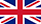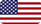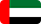• Language10:3705:3713:37

IGCSE MathematicsInstitute Name : Online Business School

Course Name : IGCSE Mathematics

Education Level : 2

Duration : 1 Year

Method of Delivery : Online

Pre Requirements : Age 14-16

Language Level : B1

Visa Type :

Application Fee :

Fees : £1500 Full

Intakes : September

Course Description :

### The Basics’

This section covers a range of topics including whole numbers, fractions, decimals, percentages, ratio, algebraic expressions, equations & formulas, coordinates & midpoints, shapes and angles, area and perimeter, collecting data, averages & range, displaying data and introduction to probability.

### Moving on (Foundation)

This module covers a range of topics including factors, multiples and primes, rounding and approximation, fractions and mixed numbers, proportion, indices, algebraic expressions, equations, formulas and inequalities, Linear sequences, graphs, shapes and angles, transformations, area and volume, compound measures, circles, displaying and interpreting data and probability.

### Preparing for Higher Tier

This module covers a range of topics including percentages, indices and standard form, algebraic expressions, equations, formulae and inequalities, non- linear sequences, graphs and functions, construction and loci, circles, cylinders, cones and spheres, similarity and congruence, Pythagoras and trigonometry, vectors, bias and sampling, grouped frequency tables and probability.

### Mastering the Higher Tier

This module covers a range of topics including decimals and place values, working with roots, algebraic expressions, quadratics, more equations and inequalities, quadratic and other non- linear sequences, graphs and functions, circle theorems, similar shapes, transformations, Pythagoras and trigonometry, vectors, inter- quartile range, histograms and probability

### Exam Preparation

This module prepares students for exams by providing exam papers for students to work through, including both Foundation and Higher Tier.